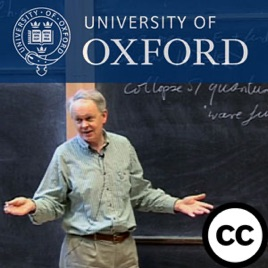27 episódios

In this series of physics lectures, Professor J.J. Binney explains how probabilities are obtained from quantum amplitudes, why they give rise to quantum interference, the concept of a complete set of amplitudes and how this defines a "quantum state". A book of the course can be obtained from http://bit.ly/binneybook# Quantum Mechanics Oxford University

• Educação

In this series of physics lectures, Professor J.J. Binney explains how probabilities are obtained from quantum amplitudes, why they give rise to quantum interference, the concept of a complete set of amplitudes and how this defines a "quantum state". A book of the course can be obtained from http://bit.ly/binneybook